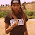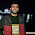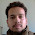## Tuesday, November 8, 2016

### Tutorial: Categorical Variational Autoencoders using Gumbel-Softmax

In this post, I discuss our recent paper, Categorical Reparameterization with Gumbel-Softmax, which introduces a simple technique for training neural networks with discrete latent variables. I'm really excited to share this because (1) I believe it will be quite useful for a variety of Machine Learning research problems, (2) this is my first published paper ever (on Arxiv, and submitted to a NIPS workshop and ICLR as well).
The TLDR; if you want categorical features in your neural nets, just let sample = softmax((logits+gumbel noise)/temperature), and then backprop as usual using your favorite automatic differentiation software (e.g. TensorFlow, Torch, Theano).

## Introduction

One of the main themes in Deep Learning is to “let the neural net figure out all the intermediate features”. For example: training convolutional neural networks results in the self-organization of a feature detector hierarchy, while Neural Turing Machines automatically “discover” copying and sorting algorithms.
The workhorse of Deep Learning is the backpropagation algorithm, which uses dynamic programming to compute parameter gradients of the network. These gradients are then used to minimize the optimization objective via gradient descent. In order for this to work, all of the layers in our neural network — i.e. our learned intermediate features — must be continuous-valued functions.
What happens if we want to learn intermediate representations that are discrete? Many "codes" we want to learn are fundamentally discrete - musical notes on a keyboard, object classes (“kitten”, “balloon”, “truck”), and quantized addresses (“index 423 in memory”).
We can use stochastic neural networks, where each layer compute the parameters of some (discrete) distribution, and its forward pass consists of taking a sample from that parametric distribution. However, the difficulty is that we can’t backpropagate through samples. As shown below, there is a stochastic node (blue circle) in between $f(z)$ and $\theta$.
Left: in continuous neural nets, you can use backprop to compute parameter gradients. Right: backpropagation is not possible through stochastic nodes.

## Gumbel-Softmax Distribution

The problem of backpropagating through stochastic nodes can be circumvented if we can re-express the sample $z \sim p_\theta(z)$, such that gradients can flow from $f(z)$ to $\theta$ without encountering stochastic nodes. For example, samples from the normal distribution $z \sim \mathcal{N}(\mu,\sigma)$ can be re-written as $z = \mu + \sigma \cdot \epsilon$, where $\epsilon \sim \mathcal{N}(0,1)$. This is also known as the “reparameterization trick”, and is commonly used to train variational autoencoders with Gaussian latent variables.

The Gumbel-Softmax distribution is reparameterizable, allowing us to avoid the stochastic node during backpropagation.
The main contribution of this work is a “reparameterization trick” for the categorical distribution. Well, not quite – it’s actually a re-parameterization trick for a distribution that we can smoothly deform into the categorical distribution. We use the Gumbel-Max trick, which provides an efficient way to draw samples $z$ from the Categorical distribution with class probabilities $\pi_i$:
$$\DeclareMathOperator*{\argmax}{arg\,max} z = \verb|one_hot|\left(\argmax_{i}{\left[ g_i + \log \pi_i \right]}\right)$$
argmax is not differentiable, so we simply use the softmax function as a continuous approximation of argmax:
$$y_i = \frac{\text{exp}((\log(\pi_i)+g_i)/\tau)}{\sum_{j=1}^k \text{exp}((\log(\pi_j)+g_j)/\tau)} \qquad \text{for } i=1, ..., k.$$
Hence, we call this the Gumbel-SoftMax distribution*. $\tau$ is a temperature parameter that allows us to control how closely samples from the Gumbel-Softmax distribution approximate those from the categorical distribution. As $\tau \to 0$, the softmax becomes an argmax and the Gumbel-Softmax distribution becomes the categorical distribution. During training, we let $\tau > 0$ to allow gradients past the sample, then gradually anneal the temperature $\tau$ (but not completely to 0, as the gradients would blow up).
Below is an interactive widget that draws samples from the Gumbel-Softmax distribution. Keep in mind that samples are vectors, and a one-hot vector (i.e. one of the elements is 1.0 and the others are 0.0) corresponds to a discrete category. Click "re-sample" to generate a new sample, and try dragging the slider and see what samples look like when the temperature $\tau$ is small!
1.0

## TensorFlow Implementation

Using this technique is extremely simple, and only requires 12 lines of Python code:

Despite its simplicity, Gumbel-Softmax works surprisingly well - we benchmarked it against other stochastic gradient estimators for a couple tasks and Gumbel-Softmax outperformed them for both Bernoulli (K=2) and Categorical (K=10) latent variables. We can also use it to train semi-supervised classification models much faster than previous approaches. See our paper for more details.

## Categorical VAE with Gumbel-Softmax

To demonstrate this technique in practice, here's a categorical variational autoencoder for MNIST, implemented in less than 100 lines of Python + TensorFlow code.
In standard Variational Autoencoders, we learn an encoding function that maps the data manifold to an isotropic Gaussian, and a decoding function that transforms it back to the sample. The data manifold is projected into a Gaussian ball; this can be hard to interpret if you are trying to learn the categorical structure within your data.
First, we declare the encoding network:

Next, we sample from the Gumbel-Softmax posterior and decode it back into our MNIST image.

Variational autoencoders minimizes reconstruction error of the data by maximizing an expectedlower bound (ELBO) on the likelihood of the data, under a generative model $p_\theta(x)$. For a derivation, see this tutorial on variational methods.
$$\log p_\theta(x) \geq \mathbb{E}_{q_\phi(y|x)}[\log p_\theta(x|y)] - KL[q_\phi(y|x)||p_\theta(y)]$$
Finally, we run train our VAE:

...and, that's it! Now we can sample randomly from our latent categorical code and decode it back into MNIST images:
Code can be found here. Thank you for reading, and let me know if you find this technique useful!

## Acknowledgements

I'm sincerely grateful to my co-authors, Shane Gu and Ben Poole for collaborating with me on this work. Shane introduced me to the Gumbel-Max trick back in August, and supplied the framework for comparing Gumbel-Softmax with existing stochastic gradient estimators. Ben suggested and implemented the semi-supervised learning aspect of the paper, did the math derivations in the Appendix, and helped me a lot with editing the paper. Finally, thanks to Vincent Vanhoucke and the Google Brain team for encouraging me to pursue this idea.
*Chris J. Maddison, Andriy Mnih, and Yee Whye Teh at Deepmind have discovered this technique independently and published their own paper on it - they call it the “Concrete Distribution”. We only found out about each other’s work right as we were submitting our papers to conferences (oops!). If you use this technique in your work, please cite both of our papers! They deserve just as much credit.

23 Apr 2017: Update - Chris Maddison and I integrated these distributions into TensorFlow's Distributions sub-framework. Here's a code example of how to implement a categorical VAE using the distributions API.

1.Could you please compare your model to model called "Discrete Variational Autoencoder" and give some thoughts on the difference and similarity of models?

https://arxiv.org/abs/1609.02200

1.2.Sure. The Discrete VAE paper (https://arxiv.org/abs/1609.02200), despite its name, is not the first paper to implement discrete variational autoencoders with stochastic discrete latent variables. Prior work includes NVIL (https://arxiv.org/pdf/1402.0030), DARN, and MuProp. Discrete VAE presents a model that counts technically as a VAE, but its forward pass is not equivalent to the model described in the other papers. In Discrete VAE, the forward sampling is autoregressive through each binary unit, which allows every discrete choice to be marginalized out in a tractable manner in the backward pass. Because the forward pass is different, the optimization objective is different, which makes it harder to compare (we are optimizing different models). The non-discrete Gumbel-Softmax relaxation also technically results in optimizing a different model as well, but since it's merely a relaxation of the original model, we can still evaluate it the same way.

Whereas DARN, MuProp, NVIL, Straight-Through Gumbel-Softmax present a way to train the same forward model, Discrete VAE optimizes a new objective altogether. It's an open question what the "right forward pass" is, but it makes it hard to compare Discrete VAE with other work since they have different forward passes and optimization strategies.

2.Great tutorial!

I am wondering what happens if the number of categories is extremely large, i.e., 1 million. Gradients need to calculated for all the \PI s and g s of a large number?

Thank you, and looking forward to hearing.

1.If categories are large, you will need a more efficient encoding of samples from the categorical distribution than one-hot vectors, otherwise you will have a rank>1e6 matrix multiply. A reparameterization trick for other encodings of vectors might be worth pursuing.

3.Hi Eric,

Agree with the posters above me -- great tutorial!

I was wondering how this would be applied to my use case: suppose I have two dense real-valued vectors, and I want to train a VAE s.t. the latent features are categorical and the original and decoded vectors are close together in terms of cosine similarity. I'm guessing that I have to change the first term of the ELBO function, since p_x.log_prob(x) isn't what I care about (is that right?). Any thoughts on what the modified version would be?

Thanks

4.I still don't see why we cannot train the same network by enforcing the latent space a one-hot vector for the example above. So if the backprop is the problem, you can flow the error through the argmax node and you can learn the parameters still. Could you give more details what is the differentiating factor of your method. Also cloud you explain what is z values on the last figure?

5.More stuff about gumbel-sigmoid here - https://github.com/yandexdataschool/gumbel_lstm/blob/master/demo_gumbel_sigmoid.ipynb

@Ero Gol
afaik, unlike max, argmax (index of maximum) will have zero/NA gradient by definition since infinitely small changes in the vector won't change index of the maximum unless there are two exactly equal elements.

6.This comment has been removed by a blog administrator.

7.Hi Eric,

I'm a student researcher at MIT doing work on the application of GAN to a discrete data set. I was wondering if there's any chance we could hop on a call so you could explain this methodology to me further?

1.Sure. Please send me an email (you can find it on my website, evjang.com)

8.p_theta(y) prior here in KL divergence code is just a categorical with equal 1/K probabilities, right?

9.Thank you Eric for this detailed introduction. Super helpful!

-Yixing

10.What are the pros and cons of using q(y|x) as discrete distribution?

11.This comment has been removed by the author.

12.Thanks Eric, this is very exciting work!

I had a question about the loss function. The latent loss here seems to be the KL-divergence between two categorical distributions (without tau). However, the density of the GS distribution in your paper involves tau. Does it simplify somehow in the KL divergence, or did you use the categorical distribution for the loss function instead?

1.